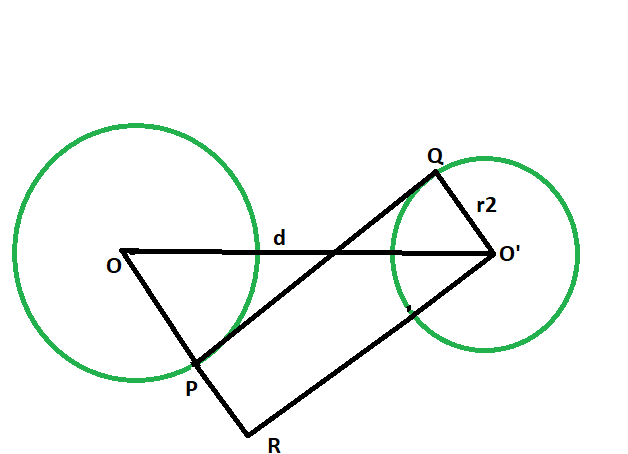# Length of the transverse common tangent between the two non intersecting circles

Given two circles of given radii, having there centres a given distance apart, such that the circles don’t touch each other. The task is to find the length of the transverse common tangent between the circles.

Examples:

```Input: r1 = 4, r2 = 6, d = 12
Output: 6.63325

Input: r1 = 7, r2 = 9, d = 21
Output: 13.6015
```Approach:

1. Let the radii of the circles be r1 & r2 respectively.
2. Let the distance between the centers be d units.
3. Draw a line O’R parallel to PQ,
4. angle OPQ = angle RPQ = 90 deg
angle O’QP = 90 deg
{ line joining the center of the circle to the point of contact makes an angle of 90 degree with the tangent }
5. angle RPQ + angle O’QP = 180 deg
PR || O’Q
6. Since opposite sides are parallel and interior angles are 90, therefore O’PQR is a rectangle.
7. O’Q = RP = r2 and PQ = O’R
8. In triangle OO’R

angle ORO’ = 90 deg
By Pythagoras theorem,
OR^2 + O’R^2 = OO’^2
O’R^2 = OO’^2 – OR^2
O’R^2 = d^2 – (r1+r2)^2
O’R^2 = √(d^2 – (r1+r2)^2)## C++

 `// C++ program to find the length ` `// of the transverse common tangent ` `// between two circles which ` `// do not touch each other ` ` `  `#include ` `using` `namespace` `std; ` ` `  `// Function to find the length ` `// of the transverse common tangent ` `void` `lengthOfTangent(``double` `r1, ``double` `r2, ``double` `d) ` `{ ` ` `  `    ``cout << ``"The length of the transverse"` `         ``<< ``" common tangent is "` `         ``<< ``sqrt``(``pow``(d, 2) - ``pow``((r1 + r2), 2)) ` `         ``<< endl; ` `} ` ` `  `// Driver code ` `int` `main() ` `{ ` `    ``double` `r1 = 4, r2 = 6, d = 12; ` `    ``lengthOfTangent(r1, r2, d); ` `    ``return` `0; ` `} `

## Java

 `// Java program to find the length ` `// of the transverse common tangent ` `// between two circles which ` `// do not touch each other ` `class` `GFG { ` ` `  `    ``// Function to find the length ` `    ``// of the transverse common tangent ` `    ``static` `void` `lengthOfTangent(``double` `r1, ` `                                ``double` `r2, ``double` `d) ` `    ``{ ` ` `  `        ``System.out.println(``"The length of the transverse"` `                           ``+ ``" common tangent is "` `                           ``+ Math.sqrt(Math.pow(d, ``2``) ` `                                       ``- Math.pow((r1 + r2), ``2``))); ` `    ``} ` ` `  `    ``// Driver code ` `    ``public` `static` `void` `main(String args[]) ` `    ``{ ` `        ``double` `r1 = ``4``, r2 = ``6``, d = ``12``; ` `        ``lengthOfTangent(r1, r2, d); ` `    ``} ` `} ` ` `  `// This code has been contributed by 29AjayKumar `

## Python3

 `# python 3 program to find the length ` `# of the transverse common tangent ` `# between two circles which ` `# do not touch each other ` `from` `math ``import` `sqrt, ``pow` ` `  `# Function to find the length ` `# of the transverse common tangent ` `def` `lengthOfTangent(r1, r2, d): ` `    ``print``(``"The length of the transverse"``, ` `                     ``"common tangent is"``,  ` `          ``'{0:.6g}'``.``format``(sqrt(``pow``(d, ``2``) ``-`  `                                ``pow``((r1 ``+` `r2), ``2``)))) ` ` `  `# Driver code ` `if` `__name__ ``=``=` `'__main__'``: ` `    ``r1 ``=` `4` `    ``r2 ``=` `6` `    ``d ``=` `12` `    ``lengthOfTangent(r1, r2, d) ` `     `  `# This code is contributed by ` `# Surendra_Gangwar `

## C#

 `// C# program to find the length ` `// of the transverse common tangent ` `// between two circles which ` `// do not touch each other ` `using` `System; ` ` `  `class` `GFG { ` `    ``// Function to find the length ` `    ``// of the transverse common tangent ` `    ``static` `void` `lengthOfTangent(``double` `r1, ` `                                ``double` `r2, ``double` `d) ` `    ``{ ` ` `  `        ``Console.WriteLine(``"The length of the transverse"` `                          ``+ ``" common tangent is "` `                          ``+ Math.Sqrt(Math.Pow(d, 2) ` `                                      ``- Math.Pow((r1 + r2), 2))); ` `    ``} ` ` `  `    ``// Driver code ` `    ``static` `public` `void` `Main() ` `    ``{ ` `        ``double` `r1 = 4, r2 = 6, d = 12; ` `        ``lengthOfTangent(r1, r2, d); ` `    ``} ` `} ` ` `  `// This code has been contributed by ajit. `

## PHP

 ` `

Output:

```The length of the transverse common tangent is 6.63325
```

Attention reader! Don’t stop learning now. Get hold of all the important DSA concepts with the DSA Self Paced Course at a student-friendly price and become industry ready.

My Personal Notes arrow_drop_upProgram Analyst Trainee,Cognizant

If you like GeeksforGeeks and would like to contribute, you can also write an article using contribute.geeksforgeeks.org or mail your article to contribute@geeksforgeeks.org. See your article appearing on the GeeksforGeeks main page and help other Geeks.

Please Improve this article if you find anything incorrect by clicking on the "Improve Article" button below.